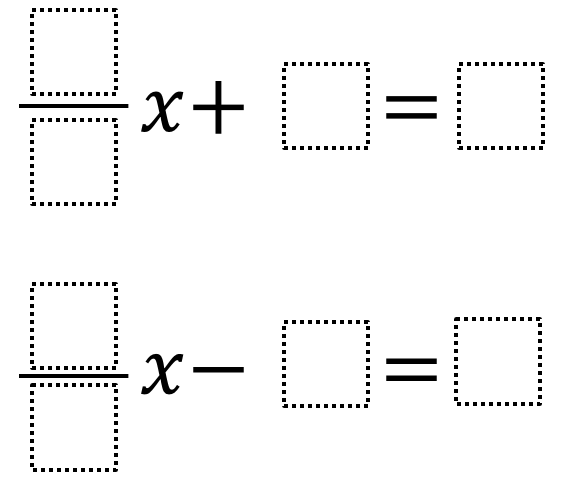Home > Grade 7 > Expressions & Equations > Exploring Equations

# Exploring Equations

Directions: For each problem below, use the digits 1 to 9 at most one time each, to fill in the boxes to find the the greatest value for x that you can.### Hint

Where should the 9 go or not go? Where should the 1 go or not go?

(1/8)x + 2 = 9 yields a solution of 56.

(1/9)x – 8 = 7 and (1/9)x – 7 = 8 both have a solution of 135.

Source:  Chase Orton and Mark Goldstein

## Sides of a Triangle

Directions: The perimeter of a triangle is 20 units. Using whole numbers, how many sets …

1.A student asked me about the value of x and since it’s on the page twice it implies they are the same value. Great activity!!

2.NO PREP! INTERACTIVE GOOGLE SLIDES that go with this problem. Link Here:
shorturl.at/ivAQW

– You can assign this to student through google classroom.
– You can use it on an interactive board/tv

•Jeffrey Galfond

Amber, How did you create those slides? Thanks so much for sharing!

•Thanks for sharing! This is awesome!